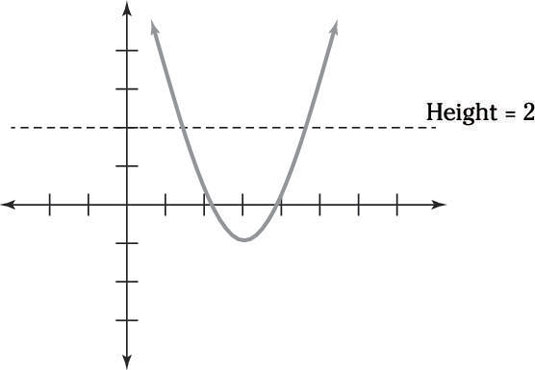##### SATYou will find some function questions on the math portion of the PSAT/NMSQT. Functions are like computers. You input something, x, and something else comes out. The variable, x, can change. (That’s why it’s called a variable!) Every time x changes, so does the result. You can express functions in different ways. You start with a long, graceful f. Then you have something in parenthesis, usually x.

This expression reads as eff of ex. Some functions look like equations:

f(x) = –3x + 8

The difference between this function and another equation is that you can plug anything into the variable spot and end up with another correct answer. In other words, the numbers in functions come in pairs. Functions can also be written as charts. Here’s a chart of some values of the preceding function: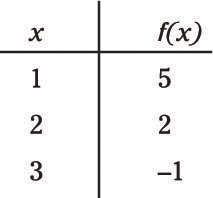A function may also appear as a graph. Here’s a graph of the preceding function: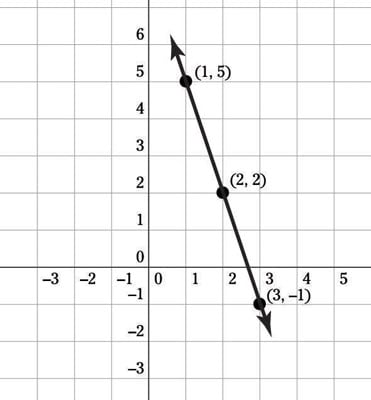For the PSAT/NMSQT, you need to get up close and personal with linear and quadratic functions. You’ve probably drawn a zillion linear functions when you created graphs. The linear function you’re most likely to run into on the exam is f(x) = mx + b.

In graphs, m is the slope of the line — the amount that the line moves forward and either up or down. The b is where the line cuts across, or intersects, the y-axis. Here’s a typical PSAT/NMSQT question about linear functions:

Which of the following charts represents a linear function?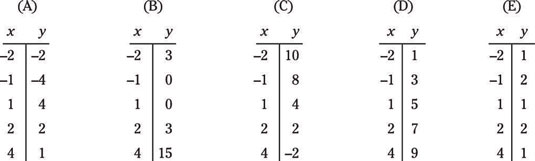First, remember that linear function really just means that the function is a straight line when you graph it, which means that it has a constant slope. One way to solve this problem is to think about the slope between each pair of points in each chart. In Choice (A), the first two points are (–2, –2) and (–1, –4).

You can think of slope as rise over run or as change in y over change in x. In this case, when x gets 1 bigger (from –2 to –1), y gets 2 smaller (from –2 to –4), meaning that the slope is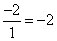Now compare the next two points, (–1, –4) and (1, 4). In this case, x gets 2 bigger and y gets 8 bigger, making a slope of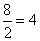Different slope, so these three points don’t make a line.

Onward to Choice (B)! The first two points, (–2, 3) and (–1, 0), have a slope of –3, and the next two points, (–1, 0) and (1, 0), have a slope of 0. Now check out Choice (C): The slope between (–2, 10) and (–1, 8) is –2 and between (–1, 8) and (1, 4) is also –2. Ooh!

Keep going to see whether –2 is the slope for the next points as well: (1, 4) and (2, 2) have a slope of –2, and (2, 2) and (4, –2) also have a slope of –2. Success! Choice (C) is the right one.

Linear functions appear in the world, too, not just on the exam. For example, the fare you pay to travel on a train may vary depending on the distance you go. In a particular city, your subway fare depends on how many stops you travel. You pay 50 cents for any ride and then an additional 25 cents for each stop that you travel.

You can model the cost of a subway ride as c = 0.50 + 0.25x, where c is the cost of the ride, and x is the number of stops that you travel.

Ready to try some function questions? Here you go:

1. Which of these points lies on the line, if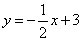(A) (–1, 5)
(B) (0, 2)
(C) (1, 3)
(D) (2, 2)
(E) (4, 2)
2. What is the equation of the line passing through the points (1, 5) and (3, 6)?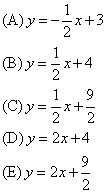1. D. (2, 2)

For each ordered pair, just plug the x-value into the x in the equation and see whether the y-value that pops out matches the y-value in the ordered pair. If it does, you found your answer! One trick here is to see that x will be divided by 2, so any odd x-value won’t have a whole number y-value paired with it, which eliminates Choices (A) and (C).

Testing Choice (B) is easy — plug in x = 0 and you get y = 3, not the y-value in the answer. In Choice (D), you plug in x = 2 and get y = 2 as the output — exactly what you’re hoping for! Choice (D) it is.

2. C.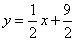Your first step should be to find the slope of the line: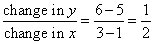which narrows your answers down to Choice (B) or (C). To determine which answer it is, plug 1 in for x and check whether the y value that pops out is 5.

Quadratic functions show up as y = ax2 + bx + c or f(x) = ax2 + bx + c. You don’t have to do much with quadratic functions, just interpret graphs. (They look like the nose of an airplane, looking down from above.)